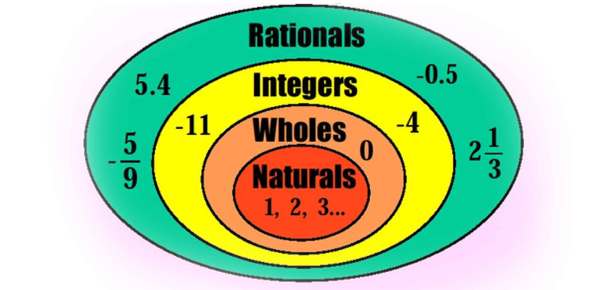# Number Systems

25 Questions | Total Attempts: 122SettingsRelated Topics
• 1.
What is the decimal value of the octal number 215?
• A.

327

• B.

141

• C.

97

• D.

100

• 2.
What is the decimal value of the hexadecimal number FF?
• A.

30

• B.

255

• C.

256

• D.

250

• 3.
Convert the sum of hexadecimal numbers 1F and 21A to binary
• 4.
What is the result of the following hexadecimal arithmetic:EA9B + FFF6
• A.

EA91

• B.

1EA91

• C.

1FA91

• D.

FA91

• 5.
Convert the binary number 1011010 to hexadecimal.
• 6.
What is the result when a decimal 5238 is converted to base 16?
• 7.
What is the equivalent of the octal number 246 in base 10?
• 8.
Digital electronics is based on the _________ numbering system.
• A.

Decimal

• B.

• C.

Binary

• D.

Octal

• 9.
Convert 1101011011 (binary) to octal
• 10.
• 11.
Find the sum of 1101012 and 10001112 and convert your result to decimal
• 12.
1001001001 (binary) to octal _____________
• 13.
• 14.
• 15.
• 16.
Find the sum of 1001012 and 11101012 and convert your result to decimal
• 17.
1111001011 (binary) to octal _____________
• 18.
• 19.
• 20.
• 21.
Find the sum of 1111012 and 11001012 and convert your result to decimal
• 22.
Evaluate the following Boolean expression:(0 + 1 + 0)(1 + 0 + 1)
• 23.
What is the binary equivalent of the hexadecimal number ABC ?
• A.

101010111100

• B.

10101011110

• C.

000001111111

• D.

100101111111

• 24.
Computers use binary digits because ________
• A.

Combinations of zeros and ones can represent any numbers and characters.

• B.

Digital devices have only two stable states

• C.

binary numbers are simple to manipulate

• D.

Binary numbers are the bases upon which all other number systems are built.

• 25.
1+1 in binary is_________________
• A.

0 carry 1

• B.

1 carry 1

• C.

1 carry 0

• D.

2 carry 0